10.13.  Energy Balance  of Fusion Processes of Molecules of Oxygen,  Hydrogen and Water

In engineering practice connected with ventilation system servicing, a phenomenon of excessive thermal energy in circulating air has been found. Similar phenomenon has been registered in water circulation systems with the devices for its active cavitations. The results of our investigations explain not only a cause of these phenomena, but they give an opportunity to perform quantitative calculations of energy processes, which generate additional thermal energy.

The atom of oxygen is the eighth element of the periodical table. The structure  of this atom  and its nucleus is shown at Fig. 61.

The least ionization energy of the electron of the oxygen atom is equal to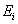=13.618 eV. Binding energy of this electron with the atomic nucleus corresponding to the first energy level is equal to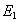=13.752 eV. The other binding energy of this electron is in Table 21.

It is known that the fusion process of the oxygen molecules is accompanied with a release of 495 kJ/mole of energy, or in calculation for one molecule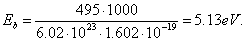(255)

What principle does the nature go by distributing energy 5.13 eV between the oxygen molecule electrons  (Fig. 67, a)? Energy of 5.13 eV is a thermal binding energy between the electrons 1 and 2’ of two oxygen atoms (Fig. 67, a). When the oxygen molecule is formed, it is emitted in the form of the photons by the electrons, which enter the bond. It appears from this that it is equal to an amount of energies of two photons emitted by these electrons. Consequently, each contacting electron emits  a photon with energies of 5.13/2=2.565 eV (Fig. 67). According to Table 20, in this case the valence electrons are situated between the second energy level and the third one.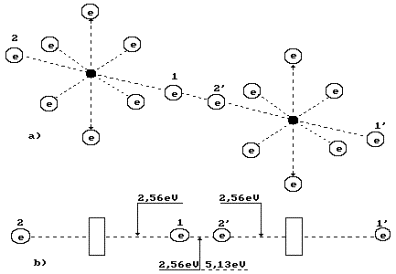Fig. 67. Diagram of binding energy distribution between the electrons in the oxygen molecule

Two oxygen atoms are connected into a molecule in an excitation state. The excitation state is the state of an atom when its valence electrons are situated at such distances from the nuclei when the binding energy between them is reduced to the thousandth fractions of an electron-volt. In such state, the atom can loose an electron and become an ion. Otherwise, without loosing electrons it is connected with an electron of the neighbouring atom by the valence electron, and a process of oxygen molecule formation begins. It is an exothermic process when the axis valence electrons 1 and 2’ emit the photons, descend on lower energy levels and release 2.565x2=5.13 eV. Let us pay attention to the fact that energy 5.13 eV is released by two electrons, which form a bond with energy of 2.56 eV. In modern chemistry, this bond is called a covalent bond. In order to break this bond, it is necessary to use 2.56 eV of mechanical energy. For thermal cleavage of this bond, double quantity of energy is required, i.e. 5.13 eV. It is explained by the fact that the photon energy of 5.13 eV is absorbed by two electrons simultaneously. Only in this case, both electrons will be transferred to the highest energy levels with minimal binding energy when they are disconnected, and each oxygen atom becomes a free one.

Thus, energy expenses for an oxygen molecule destruction depend on an impact method on the bond. During thermal impact on the bond it is destroyed when energy is 5.13 eV. During mechanical impact of the bond, it is necessary to spend 2.56 eV of energy in order to destroy this bond. It appears from this that energetic of fusion process of the oxygen molecule depends on its destruction method.

After the thermal destruction of the oxygen molecule its formation process begins from emission of the photons with energies of 2.56 eV by both valence electrons, and the previous electrodynamics binding energy (2.56 eV) is restored between the electrons of both atoms.

Thus, during the thermal destruction of the oxygen molecule the same amount of thermal energy is spent, which is released during its further formation. No additional energy appears during thermal dissociation of the oxygen molecule and its further fusion.

If the oxygen molecule is destroyed by a mechanical method, it is necessary to spend 2.56 eV of mechanical energy for this purpose. Valence electrons of the oxygen atoms are in a free state by a lack of energy corresponding to such state as there is no absorption process of 2.56 eV of energy by each of them. The electrons cannot remain in such state, they should replenish immediately the energy, which they have failed to receive during a mechanical break of the bond between them. Where should they take it? There is only one source: environment, i.e. physical vacuum filled with ether. They convert ether into energy of 2.56 eV immediately.

The next stage is a connection of two oxygen atoms, which valence electrons have replenished the reserves of their energy at the expense of ether. This process is accompanied by emission of the photons with energies of 2.56 eV by two electrons. Thus, energy of absorbed ether is converted into thermal energy of the photons. If we spend 2.56 eV of mechanical energy for the oxygen molecule destruction, we’ll get double quantity of energy (2.56x2=5.13) eV during further fusion of this molecule. Additional energy is equal to 2.56 eV.

Many experimental data show that in ventilation systems thermal energy of circulating air exceeds electric energy spent for a fan drive. Now we know that this energy is generated at mechanical failure of covalent bonds in the molecules of the gases, which the air consists of.

Using the above-mentioned method, we’ll analyse water molecule energetic, which sometimes generates additional thermal energy. A water molecule consists of one oxygen atom and two hydrogen atoms. Binding energies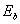of the hydrogen atoms with its nucleus are given in Table 4.

It is known that a connection of hydrogen with oxygen is accompanied by an explosion, but its cause remains unknown. Let us try to find it.

Hydrogen molecule fusion energy is equal to 436 kJ/mole, or 4.53 eV per molecule. As the molecule consists of two atoms, the above-mentioned energy is distributed between them. Thus, energy of one bond between the hydrogen atoms is equal to 2.26 eV (Fig. 68). At thermal failure of this bond, double quantity is required 2.26x2=4.53 eV.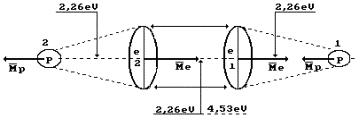Fig. 68. Hydrogen molecule

In order to form two water molecules, it is necessary to break two hydrogen molecules and one oxygen molecule into atoms. If the destruction processes of the above-mentioned molecules are carried out with a thermal method, 4.53+4.53=9.06 eV are required for the destruction of two hydrogen molecules, and 5.13 eV are required for the destruction of one oxygen molecule. Totally, it is 14.19 eV.

It is known that during fusion of one mole of water 285.8 kJ or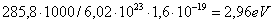per molecule are released. As a water molecule consists of one oxygen atom and two hydrogen atoms, 2.96/2=1.48 eV falls per bond (Fig. 69). It appears from this that the electrons of the atoms of hydrogen and oxygen in the water molecule are at the usual temperature 1.48/2=0.74 eV between the forth energy level and the fifth one (Table 4 and 21).

Thus, when two hydrogen molecules and one oxygen molecule are destroyed by the thermal method, 14.19 eV are spent. As a result of fusion of two water molecules, 2.96x2=5.98 eV are released. It conflicts with the fact that water molecule fusion process is an exothermic one with a release of 2.96 eV by one molecule. The given calculation shows that (14.19-5.98)/2=4.10 eV are absorbed during fusion of one water molecule. What is the cause of this contradiction?

The oxygen atom in the water molecule should reduce its volume when the transition from gaseous state into liquid state takes place. It will happen when the rings electrons of the oxygen atom descend on lower energy levels (nearer to the nucleus). They will emit the photons, and we know their total energy. It is equal to energy spent to destruction of two hydrogen molecules and one oxygen molecule, i.e. 14.19 eV. As two water molecules have 12 ring electrons, each of them will emit 14.19/12=1.18 eV (Fig. 69). It is more than axis electron binding energy (0.74 eV) with the nucleus, and it shows that the ring electrons are situated nearer to the nucleus than the axis ones.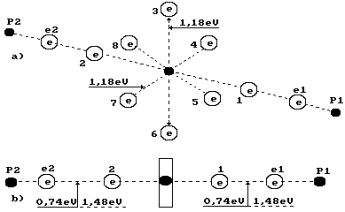Fig. 69. Diagram of water molecule:

1,2,3,4,5,6,7,8 are the numbers of the electrons of the oxygen atom; P1, P2 are the nuclei of the hydrogen atoms (the protons); e1 and e2 are the numbers of the electrons of the hydrogen atoms

In this case, quantity of the energy produced due to fusion of two water molecules (14.19+5.98) eV exceeds the energy spent for the destruction of two hydrogen molecules (9.06 eV) and one oxygen molecule (5.13 eV). The formed energy difference of 5.98 eV is divided between two water molecules. It means that 5.98/2=2.99 eV or 285.8 kJ/mole fall per molecule. It corresponds to the existing experimental data completely.

The above-mentioned facts clarify a cause of the explosion, which takes place when hydrogen is combined with oxygen. Simultaneous transition of six ring electrons of each oxygen atom in the nascent water molecules to lower energy levels is accompanied by simultaneous emission of the photons, which generate explosion phenomenon.

Let us pay attention to the fact that two binding energies between valence electrons e2 and 2 and between 1 and e1 are shown in Fig. 69, b. Energy of one electrodynamics bond is equal to 0.74 eV. If this bond is destroyed by the thermal method, 0.74x2=1.48 eV is required. This energy will be released during further fusion of the water molecule from hydrogen atom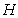and hydroxyl ion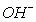. In this case, no additional energy is generated.

It appears from this that the given bond is destroyed by the mechanical method spending 0.74 eV per bond, each electron will have energy deficit equal to 0.74 eV after bond failure. This energy will be absorbed from the environment immediately and will be emitted during the repeated fusion of the water molecule from the hydrogen atom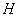and the hydroxyl ion. At mechanical failure of one bond of water molecule, the covalent chemical bond forms 0.74 eV of additional thermal energy, which is registered in the water cavitation systems constantly (as we have already noted).

It is known that the water molecules unite and form clusters. If the bonds between the molecules in the clusters are covalent ones, mechanical destruction of these bonds should be accompanied by a release of additional thermal energy as well.

In the Russian market, three firms (Yusmar, Termovikhr and Noteka) sell cavitation water heating equipment with energy efficiency index of 150%. Soon, an air heating device with the same efficiency will be produced. The processes of mechanical destruction of covalent bonds of the air gas molecules, molecules and clusters of water and their further fusion serve as a source of additional energy generated by these devices.

Physical vacuum serves as a source of additional energy generated by these heating elements. The electrons of the clusters and molecules forming covalent bonds extract this energy from physical vacuum after mechanical destruction of these bonds and release it during further fusion of the molecules and the clusters.

10.14. Energetics of Chemical Bonds of the Ozone Molecule

Ozone is a gaseous substance, which consists of three-atom molecules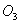.  In order to destroy the oxygen molecule, it is necessary to spend 5.13 eV of energy. During fusion of two ozone molecule, 2.99 eV of energy are released. As a result, energy difference  5.13-2.99=2.15  eV takes place. The authors of the fundamental monograph  devoted to ozone assert that energy of 2.15 eV is absorbed by the third unknown particle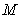, which takes part in this process. The oxygen atom, the molecules of oxygen and ozone as well as any other molecule being present in the ozone molecule fusion zone can play the role of this particle. Such assumption is made for the purpose that the law of conservation of energy will not be violated. The ozone molecule fusion reaction is written in such a way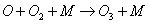.

At any rate, it is a strange assumption. It is known that each portion of energy has its owner in the processes of fusion and dissociation of the molecules. That’s why it is necessary to find a true owner of energy of  2.15 eV .

Prior to the analysis of energetics of the chemical bonds of the ozone molecule, it is necessary to understand energetics of the chemical bonds of the atom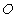and the oxygen molecule.

The oxygen molecule structure is given in Fig. 67, a. It is formed by means of a connection of unlike magnetic poles of axis electrons of two oxygen atoms . As it is clear, the oxygen molecule has fourteen free electrons, which are ready for bond. The axial electrons 1’ and 2 are the most remote ones from the structure of the whole molecule; they have the greatest activity, i.e. aptitude for bond with the electrons of other atoms .

It is known that the fusion process of the oxygen molecules is accompanied with a release of 495 kJ/mole of energy, or in calculation for one molecule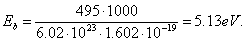(4)

What principle does the nature go by distributing energy 5.13 eV between the oxygen molecule electrons  (Fig. 67, b)? Energy of 5.13 eV is a thermal binding energy between the electrons 1 and 2’ of two oxygen atoms (Fig. 67, a). When the oxygen molecule is formed, it is emitted in the form of the photons by the electrons, which enter the bond. It appears from this that it is equal to an amount of energies of two photons emitted by these electrons. Consequently, each contacting electron emits a photon with energies of 5.13/2=2.565 eV (Fig. 67). According to Table 21, in this case the valence electrons are situated between the second energy level and the third one .

Two oxygen atoms are connected into a molecule in an excitation state. The excitation state is the state of an atom when its valence electrons are situated at such distances from the nuclei when the binding energy between them is reduced to the thousandth fractions of an electron-volt. In such state, the atom can loose an electron and become an ion. Otherwise, without loosing electrons it is connected with an electron of the neighbouring atom by the valence electron, and a process of oxygen molecule formation begins. It is an exothermic process when the axis valence electrons 1 and 2’ emit the photons, descend on lower energy levels and release 2.565x2=5.13 eV.

In order to destroy the oxygen molecule and to form the ozone molecule, spark discharge or photon flux with energies, which are somewhat larger than binding energy of 2.565х2=5.13 eV between the oxygen atoms in its molecule, are used (Fig. 67, b). It is known that ozone is formed during ultraviolet radiation with the wavelength of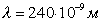. Photon energy, which corresponds to this wave length, is equal to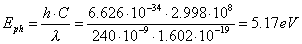As ozone is formed according to equation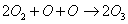,  it is necessary to destroy one oxygen molecule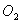for fusion of two molecules of ozone. To this effect, it is necessary to excite 2 electrons having spent 2.565x2=5.13 eV for this purpose (Fig. 67).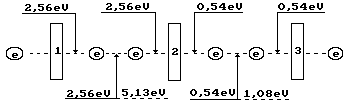Fig, 70. Diagram of binding energy distribution in the ozone moleculeIt is known that 144 kJ are released during dissociation one mole of ozone. As a result, we have per molecule: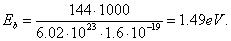(256)

Ozone formation process begins when even the smallest temperature reduction takes place in ozone where the oxygen atoms are in excited state. Their valence electrons are connected with the valence electrons of the oxygen atoms in its molecules and emit the photons with such total energy that the remainder of energy absorbed earlier (5.13 eV) will be equal to endothermic energy of 1.49x2=2.99 eV of formation of two ozone molecule. Energy of the emitted photons will be equal to 5.13-2.99=2.15 eV. This energy is spent for formation of the bonds in two ozone molecules, which have 4 valence electrons. Binding energy corresponding to one electron is equal to 2.15/4=0.54 eV (Fig. 70). In this case, valence electrons are almost on the fifth energy levels (Table 21).

As it is clear (Fig. 70), the ozone molecule is longer than the oxygen molecule (Fig. 67); binding energy (0.54 eV) between the third one, which is connected by the oxygen atom, is fivefold less than between the oxygen atoms (2.56 eV) in its molecule. As a result, stability of the ozone molecule is less than stability of the oxygen molecule, and it is destroyed easier forming the oxygen molecules and its atoms. For this effect, availability of light photons, which energy is changed within the range of 0.016-3.27 eV, is enough (Table 34).

Table 34. Electromagnetic emission scale ranges

 Bands Wave-length, m Energy, eV 1. Low- frequency band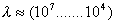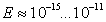2. Broadcast band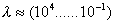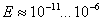3. Microwave band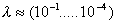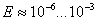4. Relic band (maximum)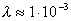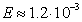5. Infrared band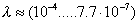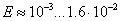6. Light band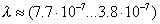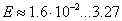7. Ultraviolet band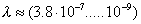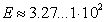8. Roentgen band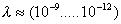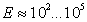9. Gamma band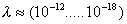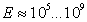After destruction of two ozone molecules, the valence electrons of the separated oxygen atoms pass into exited state absorbing 0.54x4=2,15 eV of energy. When they reach the highest energy levels, they are separated; after the free state stage, they form an oxygen molecule emitting the photons with total energy equal to 5.13 eV. Difference between emitted energy of 5.13 eV and energy of 2.15 eV absorbed by four electrons is equal to the dissociation energy of  two ozone molecule of  2.99 eV, or 1.44 kJ/mole.

The disclosed models of the oxygen atom and molecule as well as empirical mathematical law of formation of the spectra of the atoms and the ions give the possibility to make a detailed analysis of energy balance of formation of the molecules of oxygen and ozone, which corresponds to the experimental data completely.

The Foundations of Physchemistry of Microworld

Ó2003 Kanarev Ph. M.

Internet Version - http://book.physchemistry.innoplaza.net

<< Back to Physchemistry Book Index